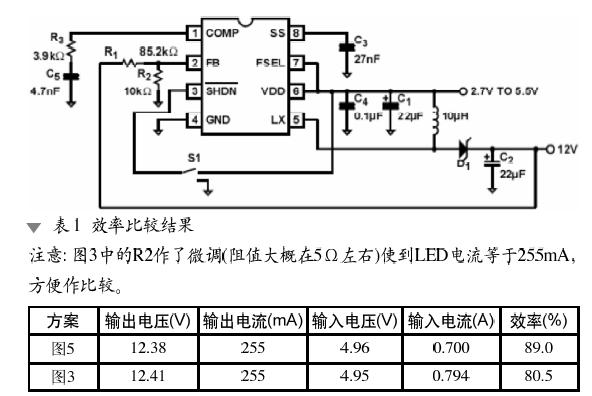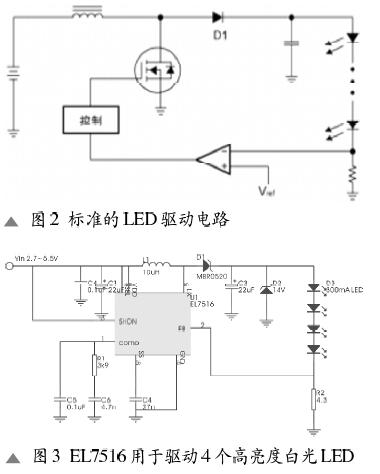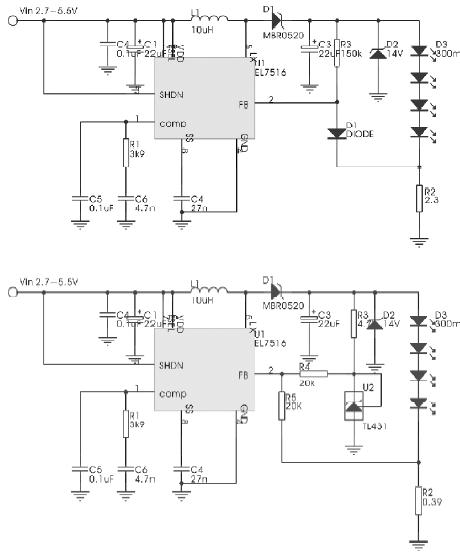# High efficiency, high current white LED driver EL7516

Foreword

With the development of white LEDs , its application has become more and more extensive. In the past, the most common application of white LEDs was as a backlight for small-size LCD color screens. Nowadays, the brightness of white LEDs has increased, and its application has spread to other aspects, such as flashlights or mobile phone camera-assisted illumination. This article describes an efficient circuit that uses a common boost chip to drive high current LEDs.

Circuit design

Generally, the current of a white LED is around 20 mA, but a high-brightness LED requires 200 to 300 mA. If the product requires three to four high-brightness white LEDs, for the average brightness, it is common practice to connect them together. Most of the white LED driver chips on the market can only drive about 20mA. If you encounter a series of high-current LED applications, you have to find another way. Intersil's EL7516 is a typical boost chip that operates in a 1.2MHz fixed-frequency PWM mode with a 1.5A, 200mÎ© MOSFET. Figure 1 shows a typical application circuit for the EL7516. The EL7516 converts the 2.7~5.5V input to a constant voltage of 12V through DC-DC boosting. Like the general PWM control chip, the FB pin is connected to an error amplifier that is compared to 1.3V. The output voltage is set by selecting the resistance values â€‹â€‹of R1 and R2.Changing the constant voltage circuit of the EL7516 to a constant current circuit that drives the LED is very simple. As shown in Figure 2, as long as R1 is replaced by an LED at the FB terminal, the current through the LED can be adjusted by changing R2. The R2 value can be selected from the following equation: R2=VFB/Iled where VFB is the voltage at the FB pin, which is 1.3V; Iled is the current through the LED.If Iled's requirement is 300mA, then R2 needs 4.3W, as shown in Figure 3.

Regarding the circuit of Figure 3, the biggest drawback is the loss of R2. When R2 passes 300mA, the power consumption of the resistor is close to 0.39W. Such large power consumption not only affects efficiency, but also requires a relatively large resistor to accept 0.39W of heat. In general, these applications are battery-powered, efficiency and circuit PCB space requirements are more stringent, now let us see how to improve the efficiency of this line.

Figure 4 shows an improvement circuit. Comparing Figure 3, Figure 4 adds two components - R3 and D1. Regardless of how the circuit is modified, the EL7516 adjusts the duty cycle to maintain the voltage to FB at 1.3V. Assume that the forward voltage drop of D1 is 0.6V, and the voltage drop of R2 is about 0.7V. To maintain a 300mA LED current, R2 can be used with 2.3Î©. The power consumption of R2 has also dropped from 0.39W to 0.21W. Although power consumption has been reduced, R2 also has to use half-watt resistors to implement this circuit.

Figure 5 shows a further improvement method. A cheap TL431 is added to the circuit as a 2.5V reference. As mentioned earlier, the EL7516 will keep the FB at 1.3V; therefore the current through R4 is: (2.5-1.3) / 20k = 60mA. Since FB is a high-impedance pin, we assume that the 60mA is all flowing into R5 and making the same 1.2V drop. The remaining 0.1V will be done by R2. For the convenience of purchase, we chose R2 as 0.39Î©. So the current through the LED is about: 0.1/0.39 = 255mA. The power consumption of R2 is also greatly reduced to: 0.1 Ã— 0.255 = 26mW. Through experiments, we obtained the efficiency comparison results of Figure 3 and Figure 5 (Table 1).Conclusion

As can be seen from the above experiments, as long as the circuit is slightly modified, the working efficiency of the high current LED can be greatly improved. In the experiment, we used 5V input. The actual application is likely to be powered by a single lithium battery. The EL7516 has a minimum operating voltage of 2.3V, so it is suitable for single lithium battery power. However, the EL7516 has a built-in MOSFET with peak current (1.3A min) protection. If the lithium battery voltage drops below 3V, driving four LEDs may trigger current protection. If you need to work below 3V, it is best to reduce the output to three LEDs.

PA66 Products

Thermal Barrier Strip,Solutions For Plastic Extrusion,Extruded Nylon Chain Guide

Room 401-2, Unit 61, Tianan Cyber City, No. 55 Changshan Road, Jiangyin City , http://www.chnonwoven.com